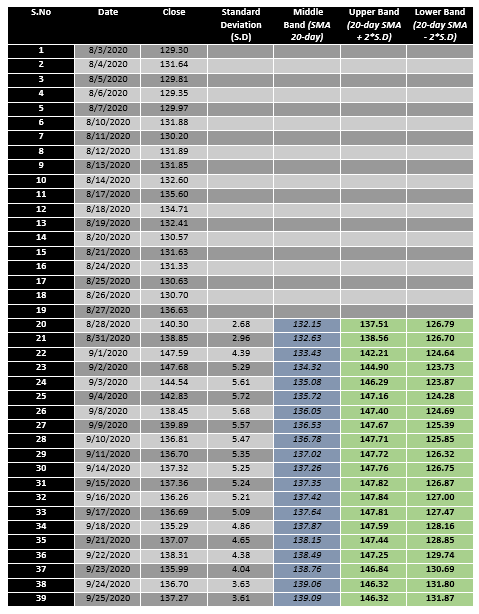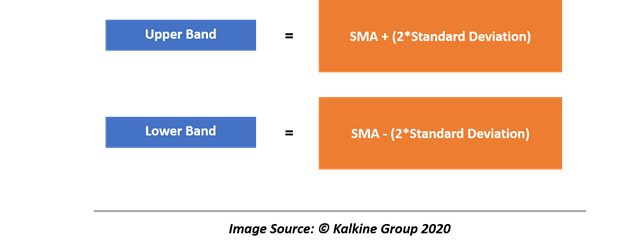# Bollinger Bands

• Updated on

## What Are Bollinger Bands®?

Originally developed by John Bollinger, Bollinger Bands are defined as the price envelope with a mean value at the centre and standard deviation at the upper and lower price range. Bollinger Bands are price envelops that are plotted at a standard deviation around a moving average that adjust to volatility swings in an underlying freely-tradable asset.

Many technical analysts use Bollinger Bands to confirm a volatility break, which further suggests large participation in the underlying asset.

Ideally, Bollinger Bands have two components, i.e., a moving average and standard deviations. The default value of Bollinger Bands used by many technical analysis software is 20, which plots a simple moving average of 20 days and plot +/-2 standard deviation around it.

To better understand the implication and derivation of Bollinger Bands, we must first understand the concept of Envelopes and Bands.

## What Are Envelopes?

Ideally, a moving average represents the centre of price action, and usually, prices tend to oscillate around a moving average. This usual move of price around a mean value or a moving average creates an envelope within which underlying security usually trades and tend to oscillate to and fro.

An envelope is usually considered as a price range above and below the mean value of a price data, in which, security tends to continue trading. However, it is not always necessary that a security would always move within an envelope around the mean value, and that is what know as an envelope breakout which technical analysts usually spot and trade.

Also, defining an envelope around a mean value could be a daunting task as there are a vast number of possible methods, tools, and techniques, which could be used to develop an envelope.

Moreover, identifying a method which best fits or justify the price fluctuations around a mean value is also challenging, and that is where Bollinger Bands come into the picture.

A Widely Used Envelop

• Percentage Envelops

One popular method of creating an envelope is to take a fixed percentage from the moving average, which is known as the percentage envelope.

For example, to create a percentage envelope, many analysts usually calculate 2% to 5% up move and down move from a mean value (depending upon the daily price change of a security) and plot the same to create an envelope around the mean value.

Furthermore, many technicians use percentage envelopes to reduce numerous unprofitable signals from crossing a moving average when the trend is sideways, allowing them to ignore any small penetration above the mean value; thus, avoiding whiplash in a sideways market.

In a percentage envelope, the percentage to plot the upper line and lower line of the envelope is often determined through experiment, and sometimes it could become very challenging to identify a percentage which would keep minimum outliers historically.

## What Are Bands?

Just like envelopes, bands are also an upper limit and lower limit plot around a mean value; however, they differ from envelopes in a way that they are dynamic rather than static and adjust for a change in the market volatility.

Thus, bands usually shrink with a decline in market volatility and expand with an increase in market volatility.

## Calculating Bollinger Bands®

For calculation purposes, let us look at the recent price data of Walmart Inc (NYSE: WMT) and try to calculate the 20-day simple Bollinger Band. The calculation of Bollinger Bands includes major components, that is, 20-day simple moving average, standard deviation, and the upper & lower limit.Although many technical analysis software such as Metastock, Amibroker, MT4, and many others provide inbuilt option to plot a Bollinger Band on a data set. The same data could be calculated using Microsoft Excel with ease.

To calculate the Bollinger Bands, we first need to calculate the standard deviation of the data (20-day if you are calculating 20-day BB). The standard deviation could be easily calculated with the function STDEVPA.

After calculating the standard deviation of the data, we need to calculate the simple moving average, which could be calculated with the AVERAGE function.

Once we have both the data we need, we could calculate the upper band and the lower band with the following formula:

##As Bolliger Bands are one type of band, their basic attributes remain as typical bands, i.e., they expand and contract to adjust for volatility. Ideally, when a Bollinger Band squeezes, it increases the likelihood of a sharp price move in either direction, which is known as the Bollinger band squeeze.

• Technicians generally spot a squeezing Bollinger Band and combine observations from other technical indicators to reach a recommendation a buy/sell/hold.

Moreover, when a price breaches the upper or lower limit of a squeezing Bollinger Band, it often regarded as an early sign of a trend reversal. However, it becomes paramount to use some confirmation to reckon if the Bollinger Band breakout (a.k.a the volatility breakout) is false or true.

• When the upper limit and the lower limit of the Bollinger Bands separate with a considerable margin, it is often linked with price extremes and considered as an early signal of a trend reversal.
• As prices usually remain within the range of a band, they show the tendency to trade from the upper range of the Bollinger Bands to the lower range, which many technicians tend to capitalise. However, this strategy, if goes wrong, could be costly and requires trailing stop losses and astute judgement.
• Many traders or technicians use the mean reversion theory and look for stocks to test the mean value of the Bollinger Band before making any recommendation.

Bolliger Band tends to expand considerably during a strong trend, and prices can breach or hug a band envelope for prolonged periods, which should be checked for divergence with momentum indicators to generate buy/sell/watch decisions.

##### Top ASX Listed Companies

Definition:
We use cookies to ensure that we give you the best experience on our website. If you continue to use this site we will assume that you are happy with it. OK